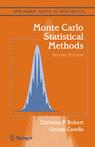## confusing errata in Monte Carlo Statistical MethodsFollowing the earlier errata on Monte Carlo Statistical Methods, I received this email from Nick Foti:

I have a quick question about example 8.2 in Monte Carlo Statistical Methods which derives a slice sampler for a truncated N(-3,1) distribution (note, the book says it is a N(3,1) distribution, but the code and derivation are for a N(-3,1)). My question is that the slice set A(t+1) is described as$\{y : f_1(y) \geq u f_1(x^{(t)}) \};$

which makes sense if u ~ U(0,1) as it corresponds to the previously described algorithm. However, in the errata for the book it says that u should actually be u(t+1) which according to the algorithm should be distributed as U(0,f1(x)). So unless something clever is being done with ratios of the f1‘s, it seems like the u(t+1) should be U(0,1) in this case, right?

There is indeed a typo in Example 8.4: the mean 3 should be -3… As for the errata, it addresses the missing time indices. Nick is right in assuming that those uniforms are indeed on (0,1), rather than on (0,f1(x)) as in Algorithm A.31. Apologies to all those confused by the errata!

This site uses Akismet to reduce spam. Learn how your comment data is processed.